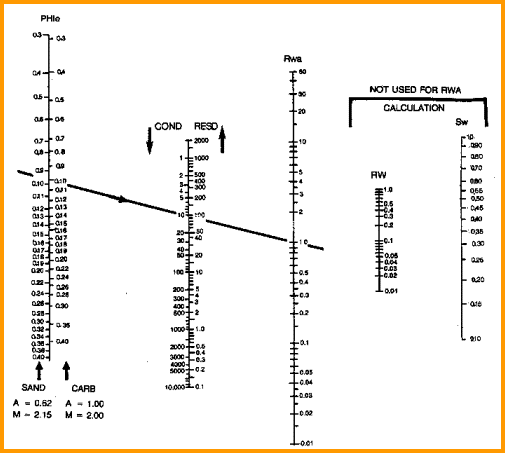Water ZONE BASICS
Back calculation of RW@FT from log data in a clean (non shaly) zone - usually called the Rwa method, or the water zone method, or the Ro (or R0) method, is commonly used when obvious water zones exist near the zone of interest.

In this method, we assume SWa = 1.00, then rearrange the Archie equation to solve for apparent water resistivity Rwa. This can be done over many relatively clean intervals and the lower Rwa values selected as RW@FT. Comparison to lab data in nearby wells or a water catalog is a useful quality control measure.RW from a Water Zone
The following algorithm is used to back calculate water resistivity from a known water zone.
1: Rwa = RW@FT = (PHIt ^ M) * RESD / A
2: Rmfa = RMF@FT = (PHIt ^ M) * RESS / A
3: Rmca = RMC@FT = 2.0 * RMF@FT

Where:
A = tortuosity exponent (unitless)
M = cementation exponent (unitless)
PHIt = total porosity found by log analysis (fractional)
RESD = deepest resistivity log reading (ohm-m)
RESS = shallowest resistivity log reading (ohm-m)
RMC@FT = mud cake resistivity at formation temperature (ohm-m)
RMF@FT = mud filtrate resistivity at formation temperature (ohm-m)
RW@FT = water resistivity at formation temperatures (ohm-m)COMMENTS:
Use this relationship if no measured values of RW are available and only if data from a clean water zone can be found. A nomographic solution is given below.

This method is often called the Rwa method

Porosity should be greater than 0.06.

Note that results are at the formation temperature. To compare these values to catalog values at 25 degrees Celsius, use the temperature transformation from the previous algorithm or the nomograph below.RECOMMENDED PARAMETERS:
for carbonates A = 1.00  M = 2.00  N = 2.00  (Archie Equation as first published)
for sandstone  A = 0.62  M = 2.15  N = 2.00  (Humble Equation)
A = 0.81  M = 2.00  N = 2.00  (Tixier Equation - simplified version of Humble Equation)
NOTE: N is often lower than 2.0

For quick analysis use carbonate values. Values for local situations should be developed from special core data. Results will always be better if good local data is used instead of traditional values, such as those given above.

Asquith (1980 page 67) quoted other authors, giving values for A and M, with N = 2.0, showing the wide range of possible values:

Average sands              A = 1.45  M = 1.54
Shaly sands                  A = 1.65  M = 1.33
Calcareous sands         A = 1.45  M = 1.70
Carbonates                   A = 0.85  M = 2.14
Pliocene sands S.Cal.  A = 2.45  M = 1.08
Miocene LA/TX             A = 1.97  M = 1.29
Clean granular             A = 1.00  M = 2.05 - PHIeWater resistivity from water zone data (Rwa Method)NUMERICAL EXAMPLE:
1. Assume data for water zone
Sand A Sand B Sand C Sand D
RESD        6 0       40        0.3         0.5
PHIt         0.33    0.14      0.30       0.11
A =  0.62
M = 2.15
RW@FT     0.89    0.94   0.036   0.007

Sample:
RW@FT = Rwa = (0.33 ^ 2.15) * 6.0 / 0.62 = 0.89

The RW@FT values represent the first approximation to a value of water resistivity for each of the four zones. The value for Sand D is not very realistic, and a better one will be found later when we look at shale corrections.

Page Views ---- Since 01 Jan 2015